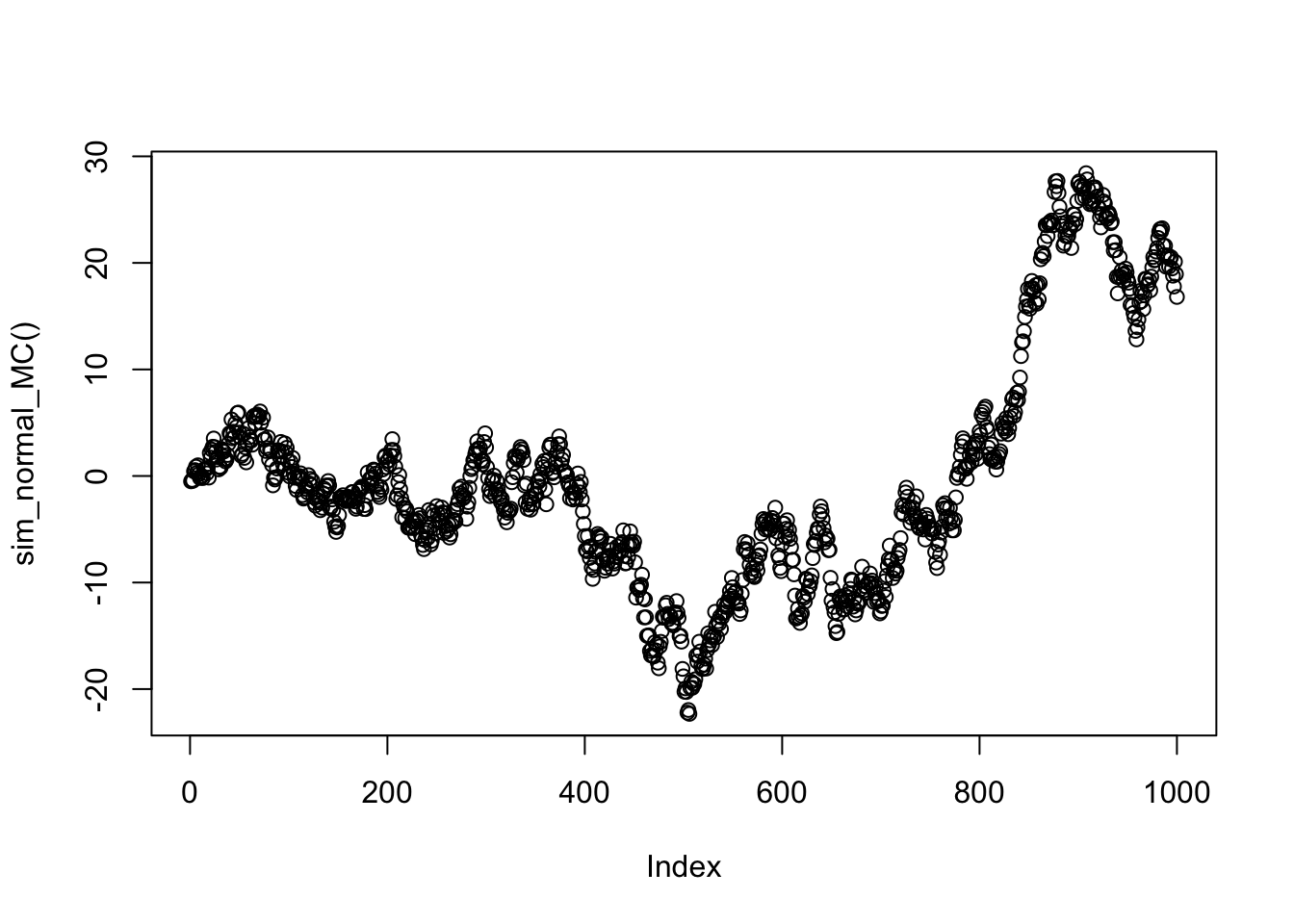Last updated: 2017-02-20

Code version: e778974f2f0b95c6ba67de64e5e5250af4abb052

# Pre-requisites

You should be familiar with the multivariate normal distribution and the idea of conditional independence, particularly as illustrated by a Markov Chain.

# Overview

This vignette introduces the precision matrix of a multivariate normal. It also illustrates its key property: the zeros of the precision matrix correspond to conditional independencies of the variables.

# Definition, and statement of key property

Let $$X$$ be multivariate normal with covariance matrix $$\Sigma$$.

The precision matrix, $$\Omega$$, is simply defined to be the inverse of the covariance matrix: $\Omega := \Sigma^{-1}$.

The key property of the precision matrix is that its zeros tell you about conditional independence. Specifically: $\Omega_{ij}=0 \text{ if and only if } X_i \text{ and } X_j \text{ are conditionally independent given all other coordinates of } X.$

It may help to compare and constrast this with the analogous property of the covariance matrix: $\Sigma_{ij}=0 \text{ if and only if } X_i \text{ and } X_j \text{ are independent}.$

That is, whereas zeros of the covariance matrix tell you about independence, zeros of the precision matrix tell you about conditional independence.

# Example: A normal markov chain

Consider a Markov chain $$X_1,X_2,X_3,\dots$$ where the transitions are given by $$X_{t+1} | X_{t} \sim N(X_{t},1)$$. You might think of this Markov chain as corresponding to a type of “random walk”: given the current state, the next state is obtained by adding a random normal with mean 0 and variance 1.

The following code simulates a realization of this Markov chain, starting from an initial state $$X_1 \sim N(0,1)$$, and plots it.

set.seed(100)
sim_normal_MC=function(length=1000){
X = rep(0,length)
X = rnorm(1)
for(t in 2:length){
X[t]= X[t-1] + rnorm(1)
}
return(X)
}
plot(sim_normal_MC())## The normal markov chain as a multivariate normal

If you think a little you should be able to see that the above random walk simulation is actually simulating from a 1000-dimensional multivariate normal distribution!

Why?

Well, let’s write each of the $$N(0,1)$$ variables we generate using ‘’rnorm’’ in that code as $$Z_1,Z_2,\dots$$. Then: $X_1 = Z_1$ $X_2 = X_1 + Z_2 = Z_1 + Z_2$ $X_3 = X_2 + Z_3 = Z_1 + Z_2 + Z_3$ etc.

So we can write $$X = AZ$$ where $$A$$ is the 1000 by 1000 matrix $A = \begin{pmatrix} 1 & 0 & 0 & 0 & \dots \\ 1 & 1 & 0 & 0 & \dots \\ 1 & 1 & 1 & 0 & \dots \\ \dots \end{pmatrix}.$

Let’s take a look at what the covariance matrix Sigma looks like. (We get a good idea from just looking at the top left corner of the matrix what the pattern is)

A = matrix(0,nrow=1000,ncol=1000)
for(i in 1:1000){
A[i,]=c(rep(1,i),rep(0,1000-i))
}
Sigma = A %*% t(A)
Sigma[1:10,1:10]
      [,1] [,2] [,3] [,4] [,5] [,6] [,7] [,8] [,9] [,10]
[1,]    1    1    1    1    1    1    1    1    1     1
[2,]    1    2    2    2    2    2    2    2    2     2
[3,]    1    2    3    3    3    3    3    3    3     3
[4,]    1    2    3    4    4    4    4    4    4     4
[5,]    1    2    3    4    5    5    5    5    5     5
[6,]    1    2    3    4    5    6    6    6    6     6
[7,]    1    2    3    4    5    6    7    7    7     7
[8,]    1    2    3    4    5    6    7    8    8     8
[9,]    1    2    3    4    5    6    7    8    9     9
[10,]    1    2    3    4    5    6    7    8    9    10

Now let us examine the precision matrix, $$\Omega$$, which recall is the inverse of $$\Sigma$$. Again we just show the top left corner of the precision matrix here.

Omega = chol2inv(chol(Sigma))
Omega[1:10,1:10]
      [,1] [,2] [,3] [,4] [,5] [,6] [,7] [,8] [,9] [,10]
[1,]    2   -1    0    0    0    0    0    0    0     0
[2,]   -1    2   -1    0    0    0    0    0    0     0
[3,]    0   -1    2   -1    0    0    0    0    0     0
[4,]    0    0   -1    2   -1    0    0    0    0     0
[5,]    0    0    0   -1    2   -1    0    0    0     0
[6,]    0    0    0    0   -1    2   -1    0    0     0
[7,]    0    0    0    0    0   -1    2   -1    0     0
[8,]    0    0    0    0    0    0   -1    2   -1     0
[9,]    0    0    0    0    0    0    0   -1    2    -1
[10,]    0    0    0    0    0    0    0    0   -1     2

Notice all the 0s in the precision matrix. This is because of the conditional independencies that occur in a Markov chain. In a Markov chain (any Markov chain) the conditional distribution of $$X_t$$ given the other $$X_s$$ ($$s \neq t$$) depends only on its neighbors $$X_{t-1}$$ and $$X_{t+1}$$. That is, $$X_{t}$$ is conditionally independent of all other $$X_s$$ given $$X_{t-1}$$ and $$X_{t+1}$$. This is exactly what we are seeing in the precision matrix above: the non-zero elements of the $$t$$th row are at coordinates $$t-1,t$$ and $$t+1$$.

## Session information

sessionInfo()
R version 3.3.2 (2016-10-31)
Platform: x86_64-apple-darwin13.4.0 (64-bit)
Running under: OS X El Capitan 10.11.6

locale:
 en_US.UTF-8/en_US.UTF-8/en_US.UTF-8/C/en_US.UTF-8/en_US.UTF-8

attached base packages:
 stats     graphics  grDevices utils     datasets  methods   base

other attached packages:
 workflowr_0.3.0 rmarkdown_1.3   ggplot2_2.2.1   dplyr_0.5.0
 magrittr_1.5    shiny_1.0.0     mixfdr_1.0      ashr_2.1.2
 dscr_0.1.1      horseshoe_0.1.0

loaded via a namespace (and not attached):
 Rcpp_0.12.9        git2r_0.18.0       plyr_1.8.4
 iterators_1.0.8    tools_3.3.2        digest_0.6.12
 memoise_1.0.0      tibble_1.2         jsonlite_1.2
 evaluate_0.10      gtable_0.2.0       lattice_0.20-34
 Matrix_1.2-7.1     foreach_1.4.3      DBI_0.5-1
 rstudioapi_0.6     curl_2.2           yaml_2.1.14
 parallel_3.3.2     httr_1.2.1         withr_1.0.2
 stringr_1.1.0      knitr_1.15.1       devtools_1.12.0
 REBayes_0.73       rprojroot_1.2      grid_3.3.2
 R6_2.2.0           EbayesThresh_1.3.2 reshape2_1.4.2
 whisker_0.3-2      backports_1.0.5    scales_0.4.1
 codetools_0.2-15   htmltools_0.3.5    MASS_7.3-45
 assertthat_0.1     mime_0.5           colorspace_1.2-7
 xtable_1.8-2       httpuv_1.3.3       labeling_0.3
 stringi_1.1.2      Rmosek_7.1.2       lazyeval_0.2.0
 munsell_0.4.3      doParallel_1.0.10  pscl_1.4.9
 truncnorm_1.0-7    SQUAREM_2016.8-2  

This site was created with R Markdown